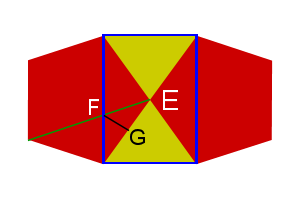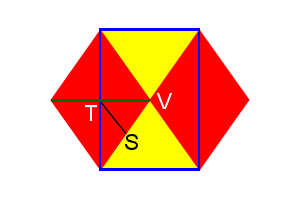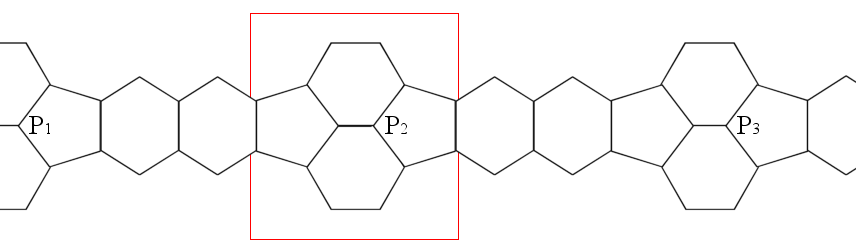# Truncated Archimedeans

In a previous post,we looked at the Platonics as portions were cut off (truncated). There are four objects that are made from further removal of edges/corners.

Unfortunately, these are not “perfect” truncations.

If you take a cuboctahedron and cut off each corner at the midpoint of each edge, you then end up with smaller triangle faces ¼th the original area and inverted and smaller squares ½ the original area and rotated 45°. You also get 12 new faces that are rectangles. This does not fit the definition of a “regular polyhedron” as rectangles are not regular polygons, they have sides that are of different lengths.

In order to get this object to be regular, you have to distort it slightly to make the rectangles into squares. Then you have a small rhombicuboctahedron. It is called this because the faces lie in the same plane as the rhombic dodecahedron, the dual of the cuboctahedron. (No one ever said that mathematicians were good at naming things.)

A better method of construction is a sort of “double truncation.” take a cube and chamfer all the edges at 45° where the cut is $$(edge length\cdot [\sqrt2-1] )$$ from each edge (Fig.1). You then truncate a second time cutting off each end of the elongated hexagons, meeting each square at the very tip of the corners, creating equilateral triangles (Fig.2).

Looking at any square face straight on, the object has the outline of a regular octagon, therefore the dihedral angle between any two squares is 135°.

Likewise, you can cut off the corners of a cuboctahedron to turn the triangle faces into hexagons and square faces into octagons, but again the new faces are rectangles, not squares. Distortion will then give you a great rhombicuboctahedron. It is also called by some a truncated cuboctahedron.

The octagon-square dihedral angle is 135°.

The white faces of both are parallel to those of an octahedron, so both can also be made from chamfering an octahedron’s edges and truncating the corners.

Another two objects can be made starting with an icosidodecahedron, instead of a cuboctahedron, the small and great rhombicosidodecahedrons and truncating appropriately.

Regardless of how you construct the objects, all faces should be regular polygons and all edges are the same length.

# Truncated Platonics

There are several Archimedean solids that are formed by the truncation (cutting off) of each corner of a Platonic solid.

These can be shown in successive truncations from one shape to its dual.

Initial truncations remove a pyramid from each corner the original object, the base of which is a regular polygon. If the original face is a triangle, the resultant edges will be $$\frac13$$ the original edge; if square, $$\sqrt\frac12$$; and if a pentagon, $$\sqrt\frac15$$.

Note: Each truncation makes the object smaller, but for the math below, the presumption is an object with edge length of 1.

Complete truncation (also called “rectified”) completely removes original edges, new faces meet a the midpoint of original edges.

Note that the left objects are “sitting” on a face, and the right objects are “standing” on a vertex. This is the result of matching the vertices of one to the midpoints of the dual.

In quasi-regular polyhedra, truncation is not necessarily resultant in regular faces. Adjustments are made to make the faces become regular, these are called rhombi-truncations.

The dihedral angles of the “main” faces (red/red or yellow/yellow) of the truncated object are the same as the original object’s dihedral angles. If there are only three faces at a vertex, the vertex angles are easily found with the formula: $$\Large{\cos\theta=\frac{\cos\epsilon}{\cos(\frac{\mu}{2})}}$$, where θ is the vertex angle of the newly created face to the opposing edge, ε is the interior angle of the new main face, and μ is the interior angle of the newly created face.

The dihedral angles of the new faces are a bit harder. We have to do each separately.

Since each truncation removes a pyramid with a regular base, we can calculate the dihedral angle of one side to the base, giving us the complimentary angle to that of the dihedral angle of the new/main faces of the polyhedra. Remember that if A+B=180, then cos A = —cos B, so we can find the cosine of the pyramid’s dihedral angle, then negate it.

I’ve already done the math for the tetrahedron, the cosine of the dihedral is $$\frac13$$, or $$70.528779\ldots^\circ$$, so the dihedral cosine for the hexagon-triangle faces of the truncated tetrahedron would be $$-\frac13$$, or $$109.47122\ldots^\circ$$. The two do add up to 180° and the latter is the same as the rectified tetrahedron (aka octahedron), therefore we are on the right track.

For the trunc. cube, a triangular based pyramid with right angles at the apex, would have a base dihedral of $$cos^{-1}(\frac{1}{\sqrt3})$$, so the octagon-triangle dihedral would be $$cos^{-1}(\frac{-1}{\sqrt3})$$, or 125.2643897…°.

The trunc. octahedron has a square pyramid (Johnson solid J1) removed that equates to a half octahedron. The dihedral for J1 is $$\cos^{-1}(\frac{1}{\sqrt3})$$, so the trunc. octahedron hex-square dihedral would be $$\cos^{-1}(\frac{-1}{\sqrt3})$$, or 125.2643897…°.

Yes, these are the same two angles, which can be seen in the cuboctahedron, all edges are square-triangles, so must be the same. It is also evident when you realize that corresponding faces are parallel from one shape to the next during truncation.

For a trunc. dodecahedron, the decagon-decagon faces have the same dihedral as the dodecahedron. The decagon-triangle edges have the same dihedral as the pent-hex edges of the trunc. icosahedron, 142.622632°, but we will check this later below.

The vertex angle θ is found by

\begin{align}\cos\theta &=\frac{\cos108}{\cos\frac{108}{2}}=\frac{-\cos72}{\cos54} \\&=-\frac{\frac{\sqrt5-1}{4}}{\sqrt{\frac{5-\sqrt5}{8}}} \\&=-\frac{\sqrt5-1}{4}\cdot\sqrt{\frac{8}{5-\sqrt5}} \\&=-\frac{\sqrt8 \cdot (\sqrt5-1)}{4\sqrt{5-\sqrt5}} \\&=-\frac{\sqrt8 \cdot \sqrt{(\sqrt5-1)^2}}{4\sqrt{5-\sqrt5}} \\&=-\frac{\sqrt8 \cdot \sqrt{6-2\sqrt5} }{4\sqrt{5-\sqrt5}} \\&=-\frac{\sqrt8 \cdot \sqrt2\cdot\sqrt{3-\sqrt5} }{4\sqrt{5-\sqrt5}} \\&=-\frac{\sqrt{16} \cdot\sqrt{3-\sqrt5} }{4\sqrt{5-\sqrt5}} \\&=-\frac{4 \cdot\sqrt{3-\sqrt5} }{4\sqrt{5-\sqrt5}} \\&=-\frac{\sqrt{3-\sqrt5} }{\sqrt{5-\sqrt5}} \\&=-\sqrt{\frac{3-\sqrt5 }{5-\sqrt5} } \\&=-\sqrt{\frac{3-\sqrt5 }{5-\sqrt5} \cdot \frac{5+\sqrt5}{5+\sqrt5} } \\&=-\sqrt{\frac{10-2\sqrt5 }{20} } \\&=-\sqrt{\frac{5-\sqrt5 }{10} } \\ \end{align}

So the vertex angle is 121.7174744…°

The trunc. icosahedron we already did the work for, the vertex angles are 148.2825255…°.

We already know the dihedrals of the cuboctahedron and icosidodecahedron are the same as their truncated counterparts, but what if we wanted to verify that?Figure 1: Icosidodecahedron vertex

As Fig. 1 shows, we can draw a rectangle onto the surface of a icosidodecahedron, with points ABCD, which creates a pyramid with apex E. As lines AD and BC cross the width of the pentagons, their length is then $$Phi\ (\phi)\ or\ \frac{1+\sqrt5}{2}$$. The green line is also Φ and crosses AD at point F. Point G is the midpoint of line DE, making DG=EG=1/2.

Notice that the blue and green lines are parallel to the upper and left sides of the pentagon, making a parallelogram, making AF=1, so $$DF=\phi-1 = \frac{\sqrt5-1}{2}$$. ∠DGF is 90°, we can find FG,

\begin{align}DG^2+FG^2 &=DF^2 \\FG^2&=DF^2-DG^2 \\&=\left(\frac{\sqrt5-1}{2}\right)^2-\frac1{2^2} \\&=\frac{6-2\sqrt5}{4} – \frac14 \\&=\frac{5-2\sqrt5}{4} \\FG&=\frac{\sqrt{5-2\sqrt5}} {2} \end{align}

The distance between F and C is

\begin{align}CF^2&=CD^2+DF^2 \\&=1+(\frac{\sqrt5-1}{2})^2 \\&=\frac44 + \frac{6-2\sqrt5}{4} \\&=\frac{10-2\sqrt5}{4} \\CF&=\frac{\sqrt{10-2\sqrt5}} {2} \end{align}

Line CG is the same as the height of the triangle, $$\frac{\sqrt3}{2}$$. Now we can find the dihedral (∠FGC)

\begin{align}\cos\angle FGC&=\frac{FG^2+CG^2-CF^2}{2\cdot FG\cdot CG} \\&=\frac{ \frac{5-2\sqrt5}{4}+\frac34-\frac{10-2\sqrt5}{4}} {2\cdot\frac{\sqrt{5-2\sqrt5}} {2}\cdot\frac{\sqrt3}{2}} \\&=\frac{ \frac{5-2\sqrt5+3-10+2\sqrt5}{4}} {(\sqrt{5-2\sqrt5})\cdot\frac{\sqrt3}{2}} \\&=\frac{-\frac24}{\sqrt{5-2\sqrt5}\cdot\frac{\sqrt3}{2}} = -\frac24\div \left(\frac{\sqrt3\sqrt{5-2\sqrt5}} {2}\right) \\&=-\frac12 \cdot \left(\frac{2}{\sqrt3\sqrt{5-2\sqrt5}}\right) \\&=\frac{-1}{\sqrt3 \sqrt{5-2\sqrt5}} \\&=\frac{-1}{\sqrt3 \sqrt{5-2\sqrt5}}\cdot \frac{\sqrt{5+2\sqrt5}}{\sqrt{5+2\sqrt5}} \\&=\frac{-\sqrt{5+2\sqrt5}}{\sqrt3 \sqrt{25-4\sqrt5-20-4\sqrt5}} \\&=\frac{-\sqrt{5+2\sqrt5}}{\sqrt3 \sqrt5} \\&=-\sqrt{\frac{5+2\sqrt5}{15} } \\\angle FGC&=142.62263\ldots^\circ \end{align}Figure 2: Cuboctahedron vertex

Just like the icosidodecahedron, we can draw a rectangle onto the surface of a cuboctahedron (Fig. 2), with points WXYZ, which creates a pyramid with apex V. As lines WZ and XY cross the diagonals of the squares, their length is then $$\sqrt2$$. The green line is also $$\sqrt2$$ and crosses WZ at point T. Point S is the midpoint of line WV, making WS=VS=1/2.

Line ST is half the width of the square, so its length is ½. Lines TV and TW are $$\frac{\sqrt2}{2}\ or \frac1{\sqrt2}$$.

The distance between T and X is

\begin{align}TX^2&=WX^2+TW^2 \\&=1+(\frac1{\sqrt2})^2 \\&=\frac22 + \frac12 \\&=\frac32 \\TX&=\sqrt{\frac32} \end{align}

Line SX is the same as the height of the triangle, $$\frac{\sqrt3}{2}$$. Now we can find the dihedral (∠TSX)

\begin{align}\cos\angle TSX&=\frac{ST^2+SX^2-TX^2}{2\cdot ST\cdot SX}=\frac{ \left(\frac12\right)^2+\left(\frac{\sqrt3}{2}\right)^2-\left(\sqrt\frac32\right)^2} {2\cdot\frac12\cdot\frac{\sqrt3}{2}} \\&=\frac{\frac14+\frac34-\frac64} {\frac{\sqrt3}{2}} \\&=\frac{-\frac24} {\frac{\sqrt3}{2}} = -\frac24 \cdot \frac2{\sqrt3} \\&=\frac{-1}{\sqrt3} \\\angle TSX&=125.2643897\ldots^\circ \end{align}

# Finding the Angles of the Truncated Icosahedron

I’ve been interested in various geometric shapes for many years, from the simple cubes, prisms, and pyramids made in middle school, to the geodesic domes used to build houses.

I have cut and glued numerous pieces of paper into just about all of the regular polyhedra.

One project I have wanted to do for some time is to build a wire-frame truncated icosahedron, otherwise known as a buckyball or the general shape of a soccer ball. Folding paper and gluing the edges where they meet is a easy task, even for kids. Gluing wood dowels or soldering wire together at specific angles tends to be a wee bit more complicated, especially if you don’t know what the angles should be.

For any of the regular polyhedra, there are hundreds of internet sites and dozens of books that will detail out how to find the dihedral angle for each face-face edge. The trouble is, wire frames don’t have “faces,” each edge joins two or more edges at a point and spread out at equal or unequal angles that are never in the same plane. I have searched high and low, but have not yet found any website that gives these angles.

I have taken the painful steps of calculating these angles for the truncated icosahedron. Using these steps you can then find the angle for any of the other regular polyhedra.

First, let’s define a few items. The truncated icosahedron is made up of 12 pentagons and 20 hexagons, all with the same side length. Since they all have the same size sides, we will define every other measurement in terms of side length, and call it 1. It can be 1 inch, 1 cm, 1 mile, or 1 light-year, it doesn’t matter.

Each pentagon is surrounded by 5 hexagons. Each hexagon is surrounded by 3 pentagons and 3 hexagons, alternating. We will call each edge where a pentagon meets a hexagon a HP edge and where 2 hexagons meet, we’ll call a HH edge. Every edge will join with two others, always an HH edge and two HP edges will meet at each vertex.

The dihedral (face to face) angle for the HH edges is given as: $$\cos^{-1}\left(\Large{-\frac{\sqrt{5}}{3}}\right) = 138.189685\ldots^\circ$$.

The dihedral angle for the HP edges is: $$\cos^{-1}\left(\Large{-\sqrt{\frac{5+2\sqrt{5}} {15} } }\right) = 142.622632\ldots^\circ$$.

As I said, there are lots of sources to find the breakdown of the math used to get these results. I only add them here for your convenience.

The diameter can be “unrolled” to show there is a repeat (Fig 1) from P1 to P2 to P3. P3 would be the same point as P1.This makes a decagon (10-sided figure), traveling across the faces of 4 pentagons, 4 hexagons, and 2 HH edges. This gives us a decagon with 4 HP angles and 2 HH angles, but we don’t know the other 4 Pentagon-to-Pentagon (Pent-Pent) edge angles.

All decagons have a total interior angle measurement of 1440°, using the formula for any N-gon, (N-2)x180. If we then use a little algebra, we get:

1440 = 4 * HP angle + 2 * HH angle + 4 * Pent-Pent edge angle

Substituting known values:

1440 = 4 x 142.622632 + 2 x 138.189685 + 4 x Pent-Pent edge angle
1440 = 570.490528 + 276.379370 + 4 x Pent-Pent edge angle
1440 = 846.869898 + 4 x Pent-Pent edge angle
1440 - 846.869898 = 4 x Pent-Pent edge angle
593.130102        = 4 x Pent-Pent edge angle
148.2825255       = Pent-Pent edge angle

So if I wish to create a wire-frame of a truncated icosahedron, then I just need to join two wires at 108°, the interior angle for a pentagon, and add a third sticking straight out and rising up at about an angle of 31¾° (180°-148¼°).

But, is this correct? How can it be verified? Is there a way to find “exact” measurements?

## Finding the Angles of a Pentagon-Hexagon-Hexagon Vertex

Taking the red-outlined area from Fig 1, and rotating we get Fig 2. Note, these figures are not to scale or proportion.

Drawing (blue) lines across the widths of the hexagons (KL & KM) and the pentagon (ML), we then get a pyramid with base ΔKLM and apex N (Fig 3).

Looking at ∠KNL and ∠KNM, each is 120° and ∠MNL=108°. The ΔNKL (Fig 4) shows how we get KL & KM from 30-60-90 triangles, each is $$2\cdot \sin60^\circ = 2·\frac{\sqrt3}{2} = \sqrt3$$.

ΔNML (Fig 5) shows the length for LM, we don’t need exact figures, 2·sin 54° will do for now. Lines GL & GM are sin 54°.

ΔKML (Fig 6) uses the Pythagorean Theorem to find length of line KG. We don’t need to find the actual length, as we are about to square it anyway.

ΔNKG (Fig 7) shows the angle η the we are looking for. Note: I drew this image even though knew that η was obtuse. 🙁

Using the Law of Cosines, we get:

\begin{align}\cos\eta &= \frac{1^2 + \color{Blue}{NG^2} – \color{LimeGreen}{KG^2}}{2\cdot1\cdot \color{Blue}{NG}}\\ &= \frac{1 + \color{Blue}{cos^2(54)} – \color{LimeGreen}{\bigl [4\cdot sin^2(60) – sin^2(54)\bigr ]}}{2\cdot1\cdot \color{Blue}{cos (54)}}\\ &= \frac{1 + \color{Blue}{cos^2(54)} \color{LimeGreen}{- 4\cdot sin^2(60)} + \color{LimeGreen}{sin^2(54)}}{2\cdot \color{Blue}{cos (54)}}\\ &= \frac{1 + \color{red}[\color{Blue}{cos^2(54)} + \color{LimeGreen}{sin^2(54)}\color{red}] \color{LimeGreen}{- 4\cdot sin^2(60)}}{2\cdot \color{Blue}{cos (54)}} & \text{Rearrange}\\ &= \frac{1 + \color{red}1 – 4\cdot sin^2(60)}{2\cdot \color{Blue}{cos (54)}} & \text{Using the identity cos² α + sin² α = 1}\\ &= \frac{2 – 4\cdot sin^2(60)}{2\cdot \color{Blue}{cos (54)}}\\ &= \frac{\color{yellow}{1 – 2\cdot sin^2(60)}}{\color{Blue}{cos (54)}} & \text{factor out 2}\\ &= \frac{\color{yellow}{\cos(2\cdot 60)}}{\color{Blue}{cos (54)}} & \text{Using the identity cos 2α = 1 – 2·sin² α}\\ &= \frac{\color{yellow}{cos(120)}}{\color{Blue}{cos(54)}}\\ &= \frac{-\frac12} {\sqrt{\frac{5-\sqrt{5}} {8} } }\\ &= \frac{-1}{2} \cdot \frac{\sqrt{8}}{\sqrt{5-\sqrt{5}}} & \text{invert}\\ &= \frac{-2\sqrt2}{2\sqrt{5-\sqrt{5}}}\cdot \frac{\sqrt{5+\sqrt{5}}}{\sqrt{5+\sqrt{5}}} & \text{Multiply by conjugate}\\ &= \frac{-\sqrt2\sqrt{5+\sqrt{5}}}{\sqrt{25-5}}\\ &= \frac{-\sqrt2\sqrt{5+\sqrt{5}}}{\sqrt{20}}\\ &= \frac{-\sqrt2\sqrt{5+\sqrt{5}}}{\sqrt{4\cdot 5}}\\ &= \frac{-\sqrt2\sqrt{5+\sqrt{5}}}{2\sqrt5}\\ &= \frac{-\sqrt2\sqrt{5+\sqrt{5}}}{2\sqrt5}\cdot \frac{\sqrt5}{\sqrt5}\\ &= \frac{-\sqrt2\sqrt5\sqrt{5+\sqrt{5}}}{20}\\ &= -\sqrt{\frac{5+\sqrt5}{10} } \end{align}
∠η = 148.2825255…°

Hooray! It matches the value we found earlier.

## Finding the Angles of a Regular Pentagonal Pyramid

A truncated icosahedron is made by truncating (cutting off) a regular pentagonal pyramid from each of the tips of an icosahedron, hence the name.

The angle of the pentagon face to any of the triangle edges will be 180° minus the Pent-Pent edge angle.

In order to find the angles we want, we must first find the height of a pentagon (Fig 8a). Either use the Pythagorean Theorem or trigonometry to find height H.

The faces of the pyramid are all equilateral triangles. Finding the triangle height S is elementary (Fig 8b). Side note: S is 1/3 the length of the original icosahedron.

Slicing through the pyramid through points A, B, and C, reveals (Fig 8c) ΔABC.

We then use the Law of Cosines to find ∠θ.

\begin{align}\cos \theta &= \frac{1^2 + \color{skyblue}{H^2} – \color{limegreen}{S^2}}{2\cdot1\cdot \color{skyblue}H}\\ &= \frac{1^2 + \color{skyblue}{\frac14\cdot tan^2(72)} \color{limegreen}{-\frac34}}{2\cdot1\cdot \color{skyblue}{\frac12\cdot tan(72)}}\\ &= \frac{\frac44 \color{limegreen}{-\frac34} + \color{skyblue}{\frac14\cdot(5+2\sqrt{5})} }{2\cdot \color{skyblue}{\frac12\sqrt{5+2\sqrt{5}}}} \\ &= \frac{\frac14+\frac14(5+2\sqrt5)}{\sqrt{5+2\sqrt5}} \\ &= \frac{1+\quad 5+2\sqrt5}{4\sqrt{5+2\sqrt5}}\\ &= \frac{6+2\sqrt5}{4\sqrt{5+2\sqrt5}}\\ &=\frac{3 + \sqrt{5}} {2\sqrt{5 + 2\sqrt{5}}}\\ &=\frac{3 + \sqrt{5}} {2\sqrt{5 + 2\sqrt{5}}} \cdot \frac{\sqrt{5 – 2\sqrt{5}}}{\sqrt{5 – 2\sqrt{5}}} & \text{multiply by conjugate}\\ &=\frac{(3 + \sqrt{5})\sqrt{5-2\sqrt5}} {2\sqrt{25-20}} \\ &=\frac{\color{yellow}{(3 + \sqrt{5})}\sqrt{5-2\sqrt5}} {2\sqrt5} \\ &=\frac{\color{yellow}{\sqrt{14 + 6\sqrt{5}}}\sqrt{5-2\sqrt5}} {2\sqrt5} & \color{yellow}{(3 + \sqrt{5})^2 = 14+6\sqrt5}\\ &=\frac{\sqrt{70 – 60 + 30\sqrt5 -28\sqrt5}} {2\sqrt5} \\ &=\frac{\sqrt{10 + 2\sqrt5}} {2\sqrt5} \\ &=\frac{\sqrt{10 + 2\sqrt5}} {2\sqrt5} \cdot \frac{\sqrt5}{\sqrt5} \\ &=\frac{\sqrt{50 + 10\sqrt5}} {10} \\ &=\frac{\sqrt{10}\sqrt{5 + \sqrt5}} {10} \\ &=\sqrt{\frac{5 + \sqrt5}{10}}\end{align}

∠θ = 31.7174744…°

θ + η = 180°

$$\cos \theta = -\cos \mu$$